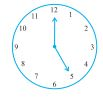Q

# The hour hand of a clock moves from 12 to 5 Is the revolution of the hour hand more than 1 right angle

The hour hand of a clock moves from 12 to 5. Is the revolution of the hour hand more than 1 right angle?Views

Yes, the revolution of the hour hand is more than 1 right angle.

For each hour, angle made =

Therefore, when the hour hand moves from 12 to 5, the angle made =

Exams
Articles
Questions